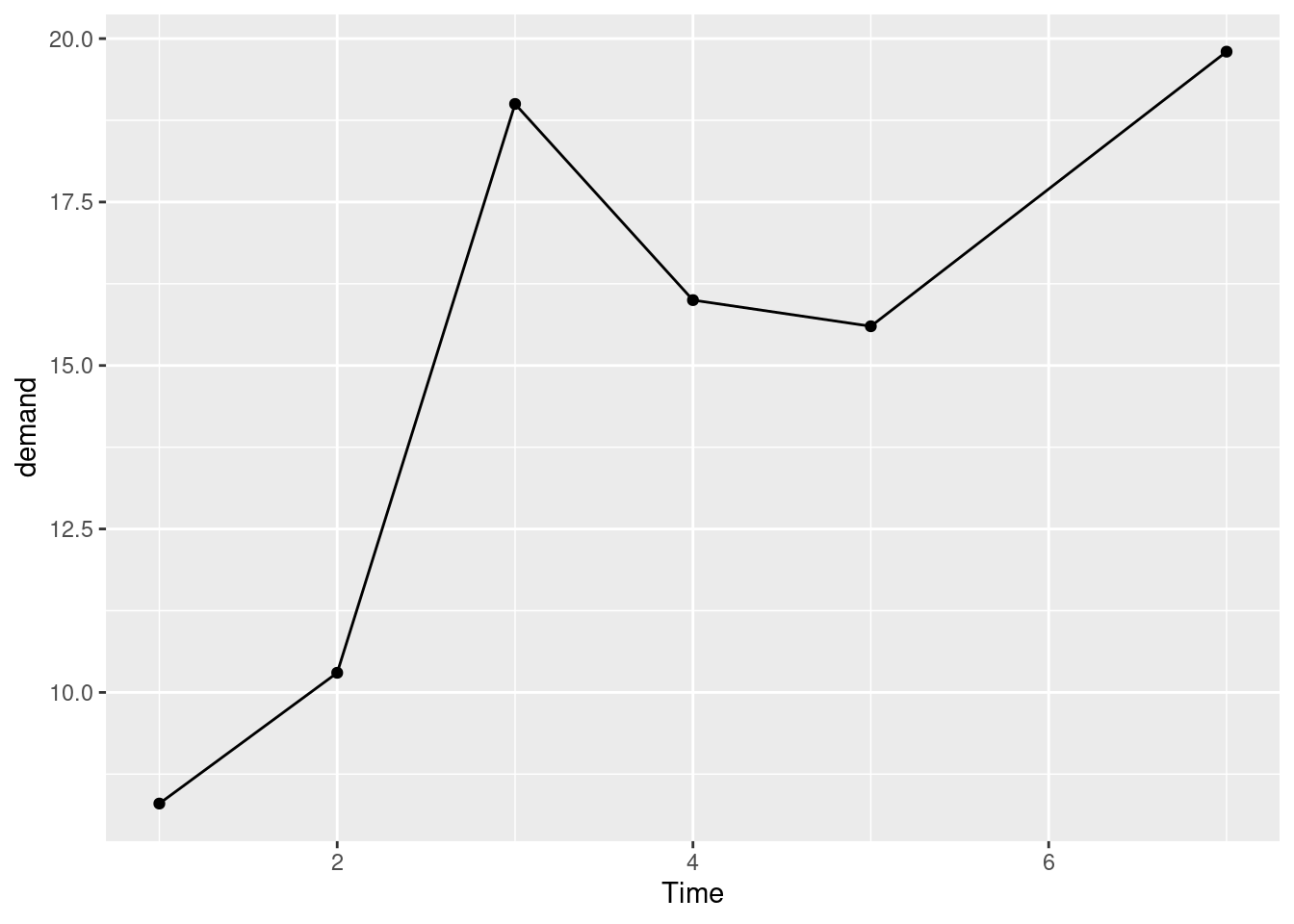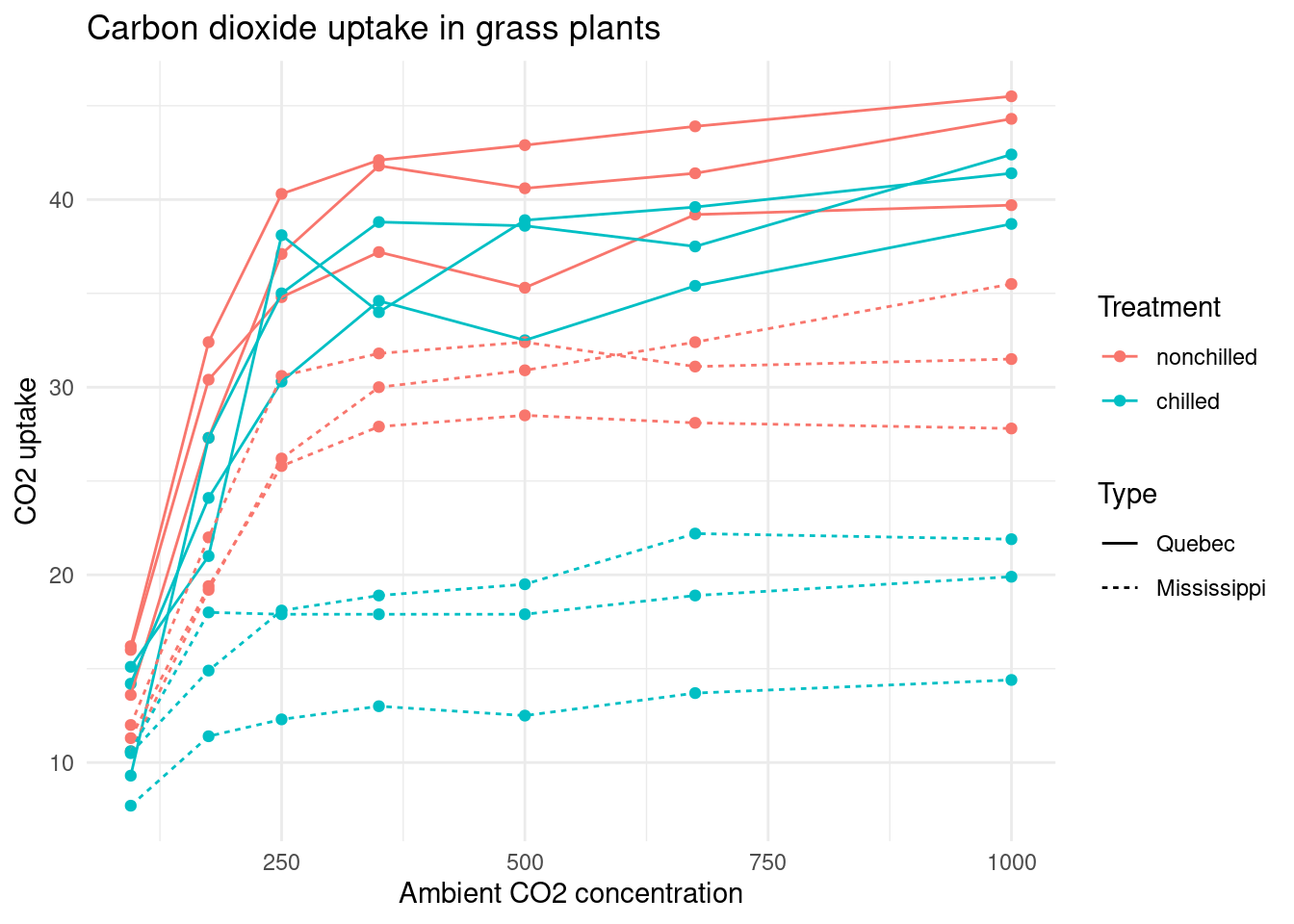## Introduction

Here are some exercises that will train you in the basics of data management and visualisation with R. In the workshop we will work through the exercises together and individually.

Each exercise involves one or more of these steps:

1. Reading data from source and assigning data to an object name.
2. Selecting columns (variables) and filtering rows (records).
3. Adding new or modifying existing variables.
4. Aggregating data, possibly after grouping by one or more categorical variables.
5. Sorting data by one or more variables.
6. Converting (pivoting) column headings to variables.
7. Plotting data.

The exercises are described in prose. The expected outputs are shown as graphs or text output. It is your job to recreate the outputs. Try to figure out how to achieve the expected output before you look at the code examples. You may show or hide code by clicking the code buttons in the right margin. Feel free to improve the output and come up with more interesting data visualisations and analyses.

NOTE: This document is dynamic and may change over time as I come up with newer and better exercises and old ones become obsolete.

## Preparing your toolbox

You will need recent versions of R (≥ 3.2) and RStudio (≥ 1.2) installed on your computer.

You also need to install the tidyverse package from within RStudio.

``install.packages('tidyverse')``

This may take several minutes.

Note that you only need to install a package once.

Check your installation by loading the tidyverse package.

``library(tidyverse)``
``## ── Attaching packages ─────────────────────────────────────── tidyverse 1.3.0 ──``
``````## ✓ ggplot2 3.3.3     ✓ purrr   0.3.4
## ✓ tibble  3.1.0     ✓ dplyr   1.0.4
## ✓ tidyr   1.1.2     ✓ stringr 1.4.0
## ✓ readr   1.4.0     ✓ forcats 0.5.1``````
``````## ── Conflicts ────────────────────────────────────────── tidyverse_conflicts() ──
## x dplyr::filter() masks stats::filter()
## x dplyr::lag()    masks stats::lag()``````

If you see messages like the ones above, everything is OK.

Note that you must load a package every time you need it.

## Visualisation intro

Make a point-and-line graph of demand vs. Time from the built-in dataset BOD.

``````ggplot(data = BOD,
aes(x = Time,
y = demand)) +
geom_line() +
geom_point()``````Make a multidimensional point-and-line graph of uptake vs. conc from the built-in dataset CO2. Map each categorical variables to their own aesthetics. Apply the “minimal theme” and add title and axis labels to the plot.

``````ggplot(data    = CO2,
mapping = aes(x        = conc,
y        = uptake,
group    = Plant,
colour   = Treatment,
linetype = Type)) +
geom_point() +
geom_line() +
theme_minimal() +
labs(title = 'Carbon dioxide uptake in grass plants',
x     = 'Ambient CO2 concentration',
y     = 'CO2 uptake')``````Same but with Type as facets.

``````theme_set(theme_minimal()) # Select minimal theme for all plots

ggplot(data    = CO2,
mapping = aes(x        = conc,
y        = uptake,
group    = Plant,
colour   = Treatment)) +
geom_point() +
geom_line() +
facet_wrap(~ Type) +
labs(title = 'Carbon dioxide uptake in grass plants',
x     = 'Ambient CO2 concentration',
y     = 'CO2 uptake')``````